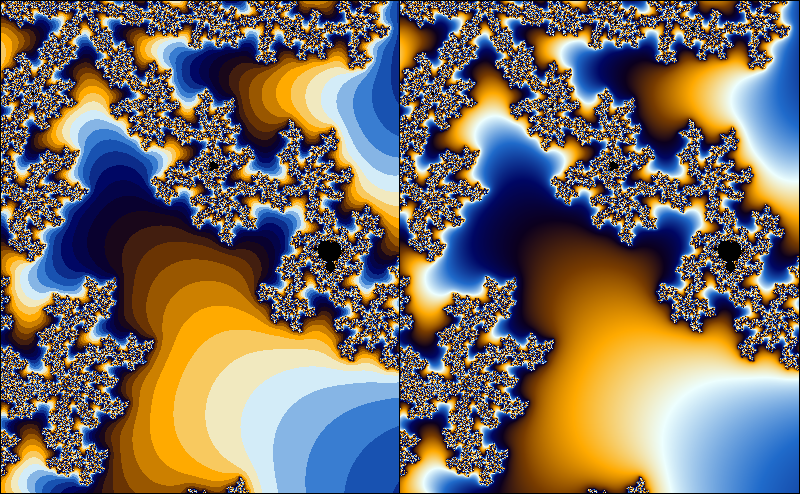# Smooth iteration count for the Mandelbrot set

The exterior of the Mandelbrot set is usually colored by using the number of iterations. As the number of iterations is a discrete quantity, this leads to the occurrence of banding in the coloring. In this article, I will derive a formula for a fractional iteration count. This is by no means novel; plenty of derivations can be found on the internet. However, I found most derivations to be fairly hard to follow, and hope that my explanation may be helpful to at least some people.## Mandelbrot formula

To check if a point is in the Mandelbrot set, we iterate

If , the set is outside the Mandelbrot set, otherwise, it is in the Mandelbrot set. In practice, we just iterate a fixed number of times, and check that stays within an escape radius . It can be shown that when for some , then . So, in a sense, is big enough. However, when we color the fractal based on the number of iterations, the escape radius matters, so in this article I'll use a general escape radius .

I will use the following conventions: the magnitude of the iterand is denoted by . The first such that for a given is called the iteration count for the point .

While the iterands (and thus, their magnitudes) depend on the starting point in a continuous way, the iteration count ‘jumps’ to discrete values. Suppose that is a very small number. A starting point for which will have an iteration count of , while a starting point with will have iteration count . If we would have some kind of measurement for the amount by which the iterand has escaped the escape radius , we could use this to assign a fractional part of the iteration count. That is, the iterand with would have an iteration count slightly lower than , while the iterand with would have an iteration count slightly higher than .

Ideally, we would like to have a smooth function such that for , and find the smallest such that . Instead of trying to find such a function, we will try to approximate it locally.

Suppose that is the smallest number such that . We then have . Assuming that is small with respect to , we get

Likewise, we find , or, more generally, . Note that this is a perfectly fine approximation even when is real. So now we can try to solve

for . In doing so, we find . Since , we have , so for the fractional iteration count we find

where is the escape radius, , and is the classical iteration count (i.e. the smallest such that ).

Exercise: a) Suppose that we instead chose to approximate at instead of . Show that we would have end up with:

b) Show that these expressions are the same. Hint: Use and .

c) Show that taking if we would take another base instead of for the inner logarithm, we would still get a smooth coloring, but if we would take another base instead of 2 for the outer logarithm, we would get a non-smooth coloring. Hint: use that .